# Bihar Board Class 10th Maths Solutions Chapter 14 Statistics Ex 14.2

Bihar Board Class 10th Maths Solutions Chapter 14 Statistics Ex 14.2 Textbook Questions and Answers.

## BSEB Bihar Board Class 10th Maths Solutions Chapter 14 Statistics Ex 14.2Question 1.
The following table shows the ages of the patients admitted in a hospital during a year :Find the mode and the mean of the data given above. Compare and interpret the two measures of central tendency.
Solution:
The class 35 – 45 has the maximum frequency. Therefore, this is the modal class.
Here l = 35, h = 10, f1 = 23, f0 = 21 and f2 = 14.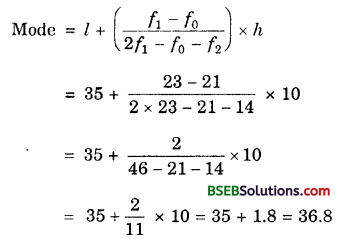Calculation of Mean
Let assumed mean, A = 30 and class size, h = 10.Hence, Mode = 36.8 years
and Mean = 35.37 years.
Maximum number of patients admitted in the hospital are of age group 36.8 years (approx,), while on an average the age of a patient admitted to the hospital is 35.37 years.

Question 2.
The following data gives the information on the observed lifetimes (in hours) of 225 electrical components :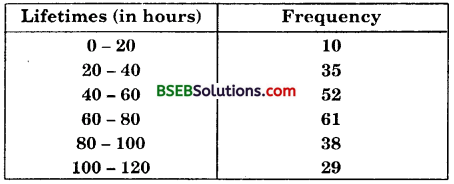Determine the modal lifetimes of the components.
Solution:
The class 60 – 80 has the maximum frequency. Therefore, this is the modal class.
Here, l = 60, h = 20, f1 = 61, f0 = 52 and f2 = 38.
Now, let us substitute these values in the formula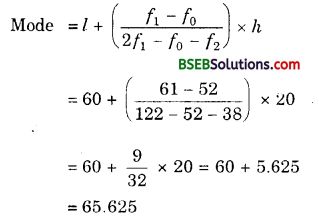Thus, the modal lifetimes of the components is 65.625 hours.

Question 3.
The following data gives the distribution of total monthly household expenditure of 200 families of a village. Find the modal monthly expenditure of the families. Also, find the mean monthly expenditure :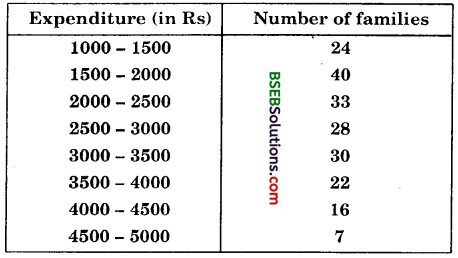Solution:
The class 1500 – 2000 has the maximum frequency. Therefore, this the modal class.
Here, l = 1500, h = 500, f1 = 40, f0 = 24 and f2 = 33.
Now, let us substitute these values in the formula∴ Modal monthly expenditure = Rs 1847.83
Let the assumed mean be A = 3250 and h = 500.Hence, the mean expenditure is Rs 2662.50.

Question 4.
The following monthly distribution gives the state-wise teacher-student ratio in higher secondary schools of India. Find the mode and mean of this data. Interpret the two measures.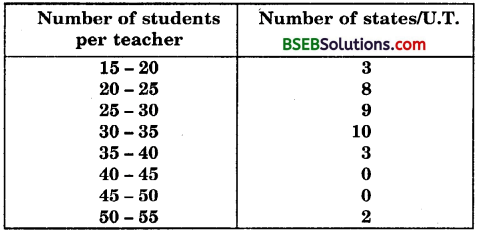Solution:
The class 30 – 35 has the maximum frequency. Therefore, this is the modal class.
Here, l = 30, h = 5, f1 = 10, f0 = 9 and f2 = 3.
Now, let us substitute these values in the formula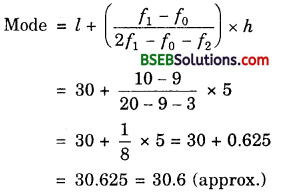Calculation of mean: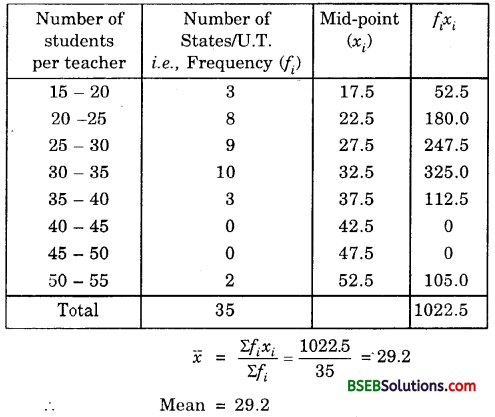Thus, most states/U.T. have a student teacher ratio of 30.6 and on an average, the ratio is 29.2.

Question 5.
The given distribution shows the number of runs scored by some top batsmen of the world in one-day international cricket matches :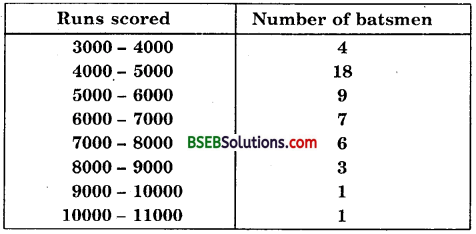Find the mode of the data.
Solution:
The class 4000 – 5000 has the maximum frequency. Therefore, this is the modal class.
Here, l = 4000, h = 1000, f1 = 18, f0 = 4 and f2 = 9.
Now, let us substitute these values in the formulaThus, the mode of the given data is 4608.7 runs.

Question 6.
A student noted the number of cars passing through a spot on a road for 100 periods each of 3 minutes and summarised it in the table given below. Find the mode of the data :Solution:
The class 40 – 50 has the maximum frequency. Therefore, this is the modal class.
Here, l = 40, h = 10, f1 = 20, f0 = 12 and f2 = 11.
Now, let us substitute these values in the formula